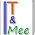## Saturday, January 16, 2010

### PCA: Dimensional Reduction in Eigen

PCA (Principal Component Analisys) is a classical machine learning method to reduce the dimensionality of a problem. PCA involves the calculation of the eigenvalue decomposition of a data covariance matrix or singular value decomposition of a data matrix, usually after mean centering the data for each attribute. Playing with Eigen library, I started to implement PCA in C++.

#### 9 comments:

1.http://www.di.unipi.it/~gulli/coding/pca_eigen.zip is correct path to the archive, didn't it?

2.yes, and the archive should be complete now.

3.Hi, I was searching around on PCA snippet/library in C++ for weeks, and I found it here it seems like the codes I needed, do you have any documentation or any quick guides on how to use it? I'm new.. Thanks a lot and appreciate your helps.. >.<

4.yes..im also need PCA code..for this year i decide to use PCA as my technique to predict the aging face...but i am not understand if in c++..could this source code of PCA in java???anyone can help me...

5.Dear Antonio,
I really appreciate your code on PCA. It is really helpful.
There is one section of the code I don't understand. This is the "// sort and get the permutation indices" section. Is this where you try to get the largest eigen value? And how do I pair up each eigen value with its corresponding eigen vector?

The out put for this section looks like this:
eigen=1.62753 pi=3
eigen=3.94939 pi=1
eigen=8.96768 pi=2
eigen=18.5922 pi=0

Thanks a lot.

6.Hi thanks a lot. It's very helpful! you know how to convert these MatrixXd VectorXd to Python without using Boost or swig?

Thanks!!

7.Thanks for the code. I prefer a much simpler solution to implement this as posted here:
http://forum.kde.org/viewtopic.php?f=74&t=110265

Works very well and is much shorter.

8.Hi, I think your implementation is wrong, instead of calculating mean of point coordinate, mean of each dimension should be calculated. The mean vector should have a length same as the number of dimension

9.Nice work. but could you direct us to the other libraries used in the code?